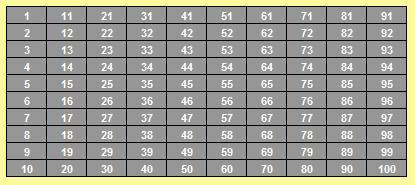# Discovering the sum of consecutive numbers

Returning home from a game of cricket on a Sunday morning, out of nowhere, a question cropped up in my mind....
What if someone asks me to mentally add up all the numbers from 1 to 100 under a minute?!
Being in the 8th grade, I did not know of any formula to add numbers from 1 to 100. So I did not have a ready answer. Luckily, I was able to derive one.
When I think of numbers, I imagine them as a two dimensional array, numbers 1-10, 11-20, 21-30 etc., forming the columns of the array.With this array in mind, I looked for some pattern in the array of 1-100 that will help me make my addition easier. Surprisingly enough, I noticed that if you traverse down the first column of the array, and traverse up the last column of the array, and add the pair of numbers, you get equal sums. For eq:
1 + 100 = 101
2 + 99 = 101
3 + 98 = 101
......
50 + 51 = 101
and so on. Having found this, now there were 50 numbers, all of then equal to 101. So the answer should be 50 x 101, which is 5050. I was happy that I was able to find the answer while I was still walking home.

Now, 100 was fairly easy. What if somebody gives me a number like 137. How do I sum 1 to 137? I found that the same travese up & traverse down rule applied. The rule was true for any number. Except that if the number is odd, you have to make an adjustment. You have to start from one number less than 137.
Eg: If you have to add numbers from 1 to 7, you have to traverse up starting from 6.
1 + 6 = 7
2 + 5 = 7
3 + 4 = 7
And then the number 7 itself.

So what's the pattern here?
For 100, the answer was 50 x 101.
For 200, the answer was 100 x 201.
For 7, the answer was 7 x 4.
For 15, the answer was 15 x 8.
I could not see the pattern instantly, but after a while, I realized
50 x 101 is nothing but 100/2 x 101.
100 x 201 is 200/2 x 201.
7 x 4 is 7 x 8/2.
15 x 8 is 15 x 16/2

At last, I had found the general pattern I was looking for...to sum up numbers from 1 to 100, you multiply, 100 & the next number 101, and then divide by 2. To add numbers from 1 to 7, you multiply 7 & the next number 8, and divide by 2.
So there goes the general rule...multiply the number with the next number & divide by 2. Back then, I did not have enough mathematical sophistication to write down the formula as n(n+1)/2, so I was content with writing down the rule in plain English words.

Later, in the 11 th grade, we came acorss the formula in the form of sigma(n) = n(n+1)/2, along with a proof using induction.

I was amused to find that there are general formulae for sum of squares and sum of cubes of consecutive numbers.
Jakob (also known as James or Jacques) Bernoulli was the first person to observe the pattern in the sums sigma(n) or summation(n), sigma(n^2), etc. Back in the year 1713, he gave a general formula to calculate sigma(n^k), which can be expressed in the form of Bernoulli numbers. To read more about Bernoulli numbers & Bernoulli polynomials, this would be a good place to start Bernoulli Numbers. This site gives a good explanation of how we can arrive at Bernoulli numbers.

A while ago, somebody asked this question on orkut.
What is the sum 1^1 + 2^2 + 3^3 + 4^4 + ... + n^n?
The solution requires use of Bernoulli polynomials, which I intend to post here in a while. Meanwhile, like to give it a try?

 Quick Comment : * Name Email ( Your email id is safe with us! ) * Comments * Type the number of characters in the word "HUMAN"